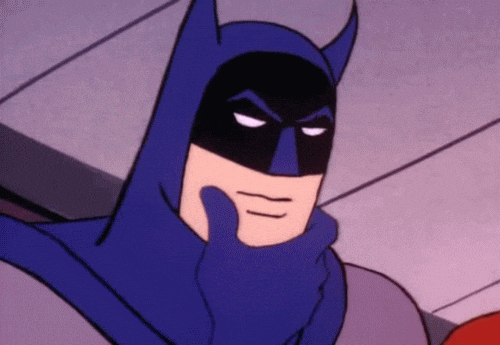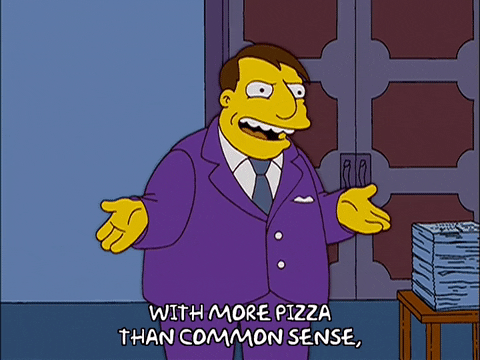Search
• Admin

# Do the Math!

Ever wonder about those people who say they are giving more than 100%? We have all been to those meetings where someone wants you to give over 100%. How about achieving 103%? What makes up 100% in life?Here's a little mathematical formula that might help you answer these questions:

If: A=1 B-2 C-3 D-4 E-5 F-6 G-7 H-8 I-9 J-10 K-11 L-12 M-13 N-14 O-15 P-16 Q-17 R-18 S-19 T-20 U-21 V-22 W-23 X-24 Y-25 Z-26 Then: H-A-R-D-W-O-R-K 8+1+18+4+23+15+ 18+11 = 98% And K-N-O-W-L-E-D-G-E 11+14+15+23+12+5+4+7+5 = 96% But , A-T-T-I-T-U-D-E 1+20+20+9+20+21+4+5 = 100% And, B-U-L-L-S-H-I-T 2+21+12+12+19+8+9+20 = 103% AND, look how far ass kissing will take you. A-S-S-K-I-S-S-I-N-G 1+19+19+11+9+19+19+9+14+7 = 118% So, one can conclude with mathematical certainty, that while Hard work and Knowledge will get you close, and Attitude will get you there. It's the Bullshit and Ass Kissing that will put you over the top. Now you know why Politicians are where they are with a score of 108!151 views0 comments

See All